# Precision Measurements of a Simple Chaotic Circuit

Ken Kiers and Dory Schmidt
Department of Physics, Taylor University, Upland, Indiana 46989

J. C. Sprott
Department of Physics, University of Wisconsin, Madison, Wisconsin 53706

(Received 10 June 2003; accepted 27 August 2003)

### ABSTRACT

We describe a simple nonlinear electrical circuit that can be used to study chaotic phenomena. The circuit employs simple electronic elements such as diodes, resistors, and operational amplifiers, and is easy to construct. A novel feature of the circuit is its use of an almost ideal nonlinear element, which is straightforward to model theoretically and leads to excellent agreement between experiment and theory. For example, comparisons of bifurcation points and power spectra give agreement to within 1%. The circuit yields a broad range of behavior and is well suited for qualitative demonstrations and as a serious research tool.

Ref: K. Kiers, D. Schmidt, and J. C. Sprott, Am. J. Phys. 72, 503-509 (2004)

The complete paper is available in PDF format.

Fig. 1. Schematic diagram of the circuit described by Eq. (6). The box labeled D(x) represents a nonlinear subcircuit. Nominal values for the unlabeled resistors and capacitors are R = 47 k  and C = 1 µF. Approximate values for the input voltage and resistor are V0 = 0.250 V and R0 = 157 k . Also, V1 =  and V2 = . The experiment employs dual LMC6062 operational amplifiers, chosen for their high input impedance. Power supplies for the operational amplifiers are tied capacitively to ground to reduce the effects of noise on the circuit.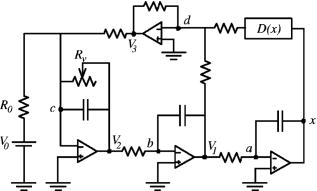Fig. 2. Schematic diagram of the subcircuit in the box in Fig. 1. The relation between the output and input voltages is given by Vout = D(Vin) = (R2/R1)min(Vin,0).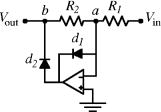Fig. 3. Experimental measurement of the function D(x) for the nonlinear subcircuit shown in Fig. 2. The superimposed line shows the function defined in Eq. (9). Both x and D(x) are in volts.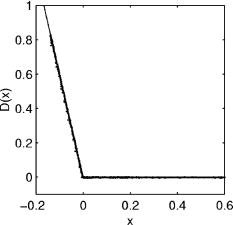Fig. 4. Experimental, theoretical, and superimposed bifurcation plots for the circuit in Figs. 1 and 2.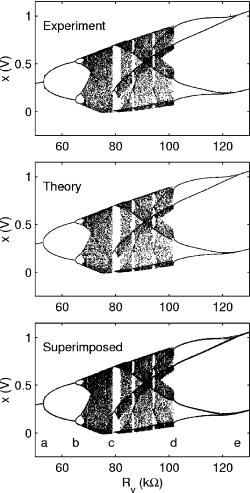Fig. 5. An expanded view of part of the experimental bifurcation plot shown in Fig. 4.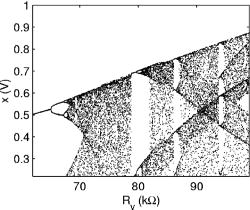Fig. 6. Experimental power spectral density plots showing the period-doubling route to chaos as Rv is increased. The value of Rv is indicated in the lower left corner. The inset in each plot shows a sample of the experimental time series data used to generate the corresponding spectral density. The smoother line in the period-one plot shows the theoretical spectral density.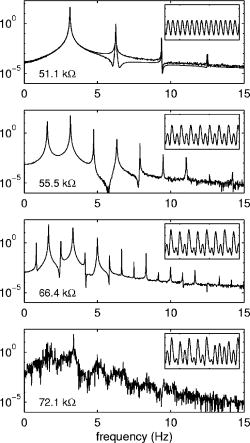Fig. 7. Experimental phase portraits for several different values of the variable resistance Rv. In each plot x and  are plotted (in volts) on the horizontal and vertical axes, respectively. A theoretical curve is superimposed on the period-six case for comparison, although the curve is not distinguishable from the experimental curve. The period-10 attractor comes from a narrow window that is barely discernible at the far right edge of Fig. 5.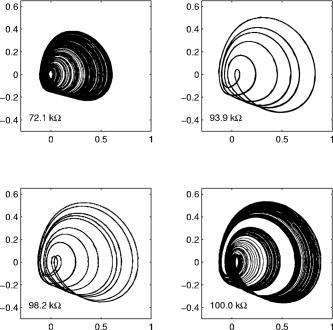Fig. 8. Stereoscopic plot of the chaotic attractor at Rv = 72.1 k . The x and  coordinates are taken from experimental data. The third coordinate is proportional to  and is determined numerically by using pairs of  values.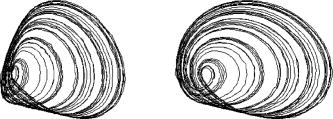Fig. 9. First- and second-return maps for Rv = 72.1 k , taken from experimental data. The insets are magnified by a factor of approximately 6.5 and show the fractal structure of the plots. The intersections of the return maps with the diagonal lines give evidence for (unstable) period-one and -two orbits in the data sets. Such orbits do indeed exist, as seen in Fig. 10.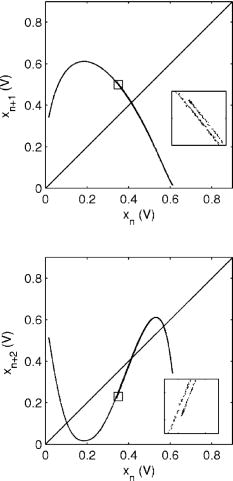Fig. 10. Experimental wave forms showing unstable period-one and -two orbits within the chaotic time series data for Rv = 72.1 k . The top plot shows an unstable period-one orbit starting shortly after t = 60 s, with a maximum near 0.41 V. The bottom plot shows an unstable period-two orbit starting just before t = 75 s, with maxima near 0.57 V and 0.10 V. The plots themselves are taken from different datasets.